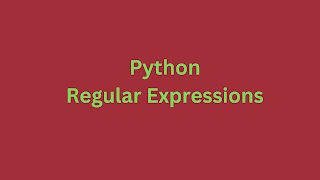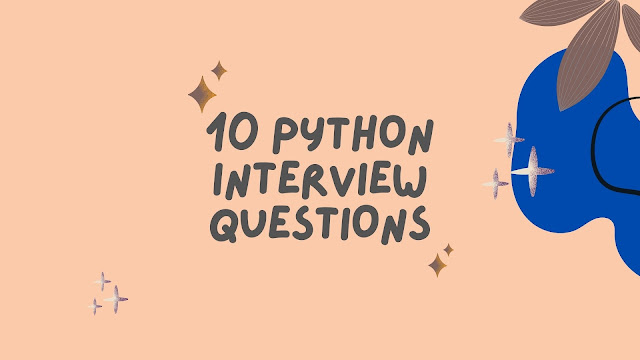### Python Regex: The 5 Exclusive ExamplesRegular expressions (regex) are powerful tools for pattern matching and text manipulation in Python. Here are five Python regex examples with explanations: 01 Matching a Simple Pattern import re text = "Hello, World!" pattern = r"Hello" result = re.search(pattern, text) if result:     print("Pattern found:", result.group()) Output: Output: Pattern found: Hello This example searches for the pattern "Hello" in the text and prints it when found. 02 Matching Multiple Patterns import re text = "The quick brown fox jumps over the lazy dog." patterns = [r"fox", r"dog"] for pattern in patterns:     if re.search(pattern, text):         print(f"Pattern '{pattern}' found.") Output: Pattern 'fox' found. Pattern 'dog' found. It searches for both "fox" and "dog" patterns in the text and prints when they are found. 03 Matching Any Digit   import re text = "The price of the

# 10 Must Read Python Interview QuestionsHere are ten python interview questions useful for your project use and other interviews.

## Python Interview questions

Python is a powerful scripting language. And you can use it in the web development, and science applications. Below, you will find interview questions on core python and web-framework as well.

1. What is PEP8?

PEP8 is a set of rules to format Python code for better readability. Further, you can read the user guide on PEP8.

2. What is memory management, and explain in detail?

Python manages memory automatically - cleaning, allocating, and managing; the detailed mechanism is as follows:

In the interview, you need to tell as below:

• Python manages private heap space and is accessible to the only interpreter.
• In heap space, Python stores objects and data structures.
• The memory allocation is the responsibility of the memory manager
• Python heap divides into pools (1 heap=64 pool), and the Pool divides into blocks (256 KB).
• Python has an inbuilt garbage collector, which recycles the unused memory and frees the memory.

3. What are the different data types?

• int
• string
• list
• tuple
• dictionary
• Boolean

Check here more on data types.

4. What is Python Copy an object?

The '=' operator and COPY module you can use to copy objects. Here are the examples.

5. What are the various types of inheritances?

There are different types of inheritances:

• Single inheritance
• Multiple-inheritance
• Multi-level inheritance
• Hierarchical inheritance
• Hybrid inheritance

Here is the example for each inheritance.

6. What are the differences between List and Tuple?

The list is mutable. But, tuple is immutable. Check out more differences between these two.

7. What is List Comprehension?

It is a concise way of creating a list and is popularly called List Comprehension.

Example:

a=[n*2 for n in range(10)]
print(a)
myList = [ num**2 for num in range(1,10) if num %2==0]
print(myList)

Python List comprehension examples.

8. What is dictionary comprehension?

The method of transforming one Dictionary into another Dictionary is called dictionary comprehension. Here are more examples of.

9. What is slicing?

You can slice an object using the slice() method, which allows three parameters - start, length, and separator you can give.

Here is the best example for the slice method.

10. What is a negative index?

Internally python assigns to '-1' for the last occurrence of a string. Python assigns a negative index (-1, -2, and so on) in reverse order.  Here is the best example for a negative index.

References